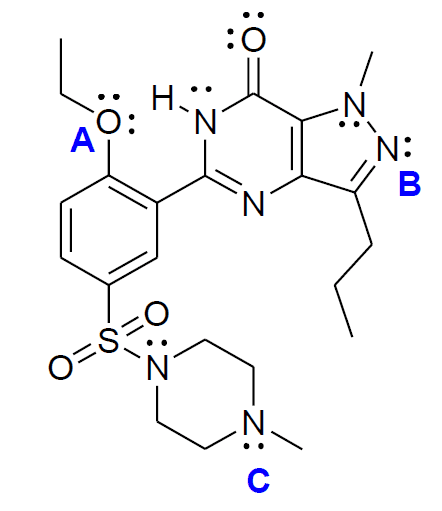# Organic chemistry hybridization and bond angles

• kirsten_2009
In summary, the hybridization of atoms A, B, and C in the molecule is sp3, sp2, and sp3 respectively. The bond angles around them are greater than 109.5 degrees, less than 120 degrees, and less than 109.5 degrees respectively.

## Homework StatementDetermine the hybridization of the atoms labeled A, B and C in the molecule and the bond angles
around them.

## Homework Equations

N/A

3. The Attempt at a Solution

A. Normally, I would say it is sp3 for oxygen since there are 4 groups attached to it including the lone pairs. However because it is attached to an sp2 carbon; the lone pair could de-localize and the charge spread. Thus I would think the O's hybridization is actually sp2. Also, I would say the bond angles are less than 109.5 (I don't know if there is an actual or absolute value) because a tetrahedral arrangement has this bond angle but the lone pairs push the bond angles closer together than a tetrahedral bond angle?

B. Since it is bonded to 3 groups including it's lone pair, and has a double bond, I would say sp2? Bond angle less than 120 degrees since it is bent but greater than 109.5 because it's not tetrahedral?

C. Sp3 since the N is attached to 4 groups including it's lone electrons and is not attached to an sp2 carbon which could de-localize any charges. Bond angles would be 109.5 because it is in a tetrahedral arrangement?

Thanks in advance for the help.

kirsten_2009 said:

## Homework Statement

View attachment 78300

Determine the hybridization of the atoms labeled A, B and C in the molecule and the bond angles
around them.

## Homework Equations

N/A

3. The Attempt at a Solution

A. Normally, I would say it is sp3 for oxygen since there are 4 groups attached to it including the lone pairs. However because it is attached to an sp2 carbon; the lone pair could de-localize and the charge spread. Thus I would think the O's hybridization is actually sp2. Also, I would say the bond angles are less than 109.5 (I don't know if there is an actual or absolute value) because a tetrahedral arrangement has this bond angle but the lone pairs push the bond angles closer together than a tetrahedral bond angle?

B. Since it is bonded to 3 groups including it's lone pair, and has a double bond, I would say sp2? Bond angle less than 120 degrees since it is bent but greater than 109.5 because it's not tetrahedral?

C. Sp3 since the N is attached to 4 groups including it's lone electrons and is not attached to an sp2 carbon which could de-localize any charges. Bond angles would be 109.5 because it is in a tetrahedral arrangement?

Thanks in advance for the help.

For atoms in the first complete row, count up the number of bonding atoms and lone pairs. This will tell you what the hybridization is.

4: sp^3; 3: sp^2, 2: sp

A: two lone pairs, and two bonded atoms. 4 => sp3 I can see how you might think that there is de-localization between the aryl ring and the O, but O's valence is filled, it cannot have a double bond going to it. C-O-C bond angle > 109.5 degrees (lone pairs on oxygen repel each other and the bonding pairs)

B: one lone pair, two bonded atoms. 3 => sp2 N-N-C bond angle < 120 degrees (lone pair repels the bonding pairs)

Cne lone pair, three bonded atoms 4 => sp3. C-N-C bond angles < 109.5 degrees (lone pair repels the bonding pairs)